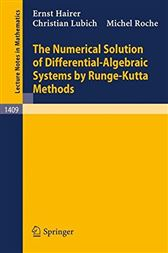# The Numerical Solution of Differential-Algebraic Systems by Runge-Kutta Methods

## Series: Lecture Notes in Mathematics (No. 1409)CA\$29.44
###### ISBNs
• 9783540518600
• 9783540468325
The term differential-algebraic equation was coined to comprise differential equations with constraints (differential equations on manifolds) and singular implicit differential equations. Such problems arise in a variety of applications, e.g. constrained mechanical systems, fluid dynamics, chemical reaction kinetics, simulation of electrical networks, and control engineering. From a more theoretical viewpoint, the study of differential-algebraic problems gives insight into the behaviour of numerical methods for stiff ordinary differential equations. These lecture notes provide a self-contained and comprehensive treatment of the numerical solution of differential-algebraic systems using Runge-Kutta methods, and also extrapolation methods. Readers are expected to have a background in the numerical treatment of ordinary differential equations. The subject is treated in its various aspects ranging from the theory through the analysis to implementation and applications.
• Springer Berlin Heidelberg; November 2006
• ISBN: 9783540468325
• Read online, or download in DRM-free PDF (digitally watermarked) format
• Title: The Numerical Solution of Differential-Algebraic Systems by Runge-Kutta Methods
• Series: Lecture Notes in Mathematics (No. 1409)
• Author: Ernst Hairer; Christian Lubich; Michel Roche
• Imprint: Springer
###### ISBNs
• 9783540518600
• 9783540468325
###### ISBNs
• 9783540518600
• 9783540468325